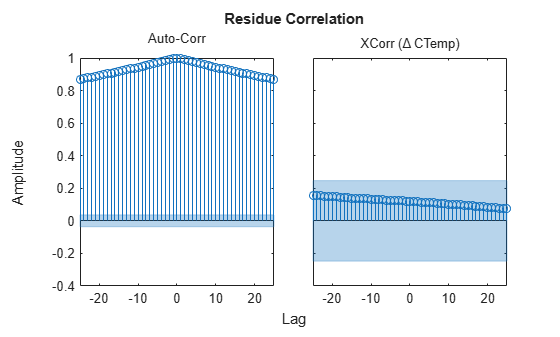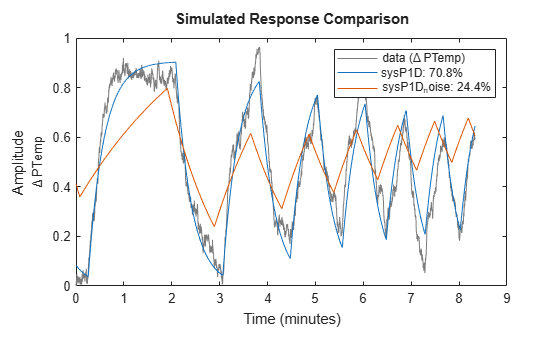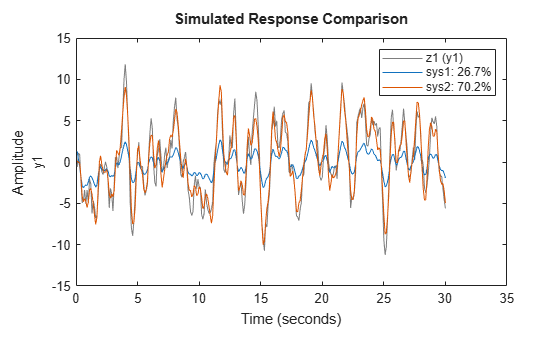procest

Estimate process model using time or frequency data

Description

example

sys = procest(data,type) estimates a process model, sys, using time or frequency-domain data, data. type defines the structure of sys.

sys = procest(data,type,'InputDelay',InputDelay) specifies the input delay InputDelay.

example

sys = procest(data,init_sys) uses the process model init_sys to configure the initial parameterization.

example

sys = procest(___,opt) specifies additional model estimation options. Use opt with any of the previous syntaxes.

example

[sys,offset] = procest(___) returns the estimated value of the offset in input signal. Input offset is automatically estimated when the model contains an integrator, or when you set the InputOffset estimation option to 'estimate' using procestOptions. Use offset with any of the previous syntaxes.

example

[sys,offset,ic] = procest(___) returns the estimated initial conditions as an initialCondition object. Use this syntax if you plan to simulate or predict the model response using the same estimation input data and then compare the response with the same estimation output data. Incorporating the initial conditions yields a better match during the first part of the simulation.

Input Arguments

collapse all

Estimation data, specified as an iddata object containing the input and output signal values, for time-domain estimation. For frequency-domain estimation, data can be one of the following:

• Recorded frequency response data (frd (Control System Toolbox) or idfrd)

• iddata object with its properties specified as follows:

• InputData — Fourier transform of the input signal

• OutputData — Fourier transform of the output signal

• Domain'Frequency'

data must have at least one input and one output.

Time-series models, which are models that contain no measured inputs, cannot be estimated using procest. Use ar, arx, or armax for time-series models instead.

Process model structure, specified for SISO models as a character vector or string representing an acronym for the model structure, such as 'P1D' or 'P2DZ'. The acronym is made up of:

• 0, 1, 2, or 3 — Number of time constants (poles) to be modeled. Possible integrations (poles in the origin) are not included in this number.

• I — Integration is enforced (self-regulating process).

• D — Time delay (dead time).

• Z — Extra numerator term, a zero.

• U — Underdamped modes (complex-valued poles) permitted. If U is not included in type, all poles must be real. The number of poles must be 2 or 3.

For MIMO models, use an Ny-by-Nu cell array of character vectors or string array, with one entry for each input-output pair. Here Ny is the number of inputs and Nu is the number of outputs.

For information regarding how type affects the structure of a process model, see idproc.

Input delays, specified as a numeric vector specifying a time delay for each input channel. Specify input delays in the time unit stored in the TimeUnit property.

For a system with Nu inputs, set InputDelay to an Nu-by-1 vector. Each entry of this vector is a numerical value that represents the input delay for the corresponding input channel. You can also set InputDelay to a scalar value to apply the same delay to all channels.

System for configuring initial parametrization of sys, specified as an idproc object. You obtain init_sys by either performing an estimation using measured data or by direct construction using idproc. The software uses the parameters and constraints defined in init_sys as the initial guess for estimating sys.

Use the Structure property of init_sys to configure initial guesses and constraints for Kp, Tp1, Tp2, Tp3, Tw, Zeta, Td, and Tz. For example:

• To specify an initial guess for the Tp1 parameter of init_sys, set init_sys.Structure.Tp1.Value as the initial guess.

• To specify constraints for the Tp2 parameter of init_sys:

• Set init_sys.Structure.Tp2.Minimum to the minimum Tp2 value.

• Set init_sys.Structure.Tp2.Maximum to the maximum Tp2 value.

• Set init_sys.Structure.Tp2.Free to indicate if Tp2 is a free parameter for estimation.

If opt is not specified, and init_sys was obtained by estimation, then the estimation options from init_sys.Report.OptionsUsed are used.

Estimation options, specified as an procestOptions option set. The estimation options include:

• Estimation objective

• Handling on initial conditions and disturbance component

• Numerical search method to be used in estimation

Output Arguments

collapse all

Identified process model, returned as an idproc model of a structure defined by type.

Information about the estimation results and options used is stored in the model's Report property. Report has the following fields:

Report FieldDescription
Status

Summary of the model status, which indicates whether the model was created by construction or obtained by estimation.

Method

Estimation command used.

InitialCondition

Handling of initial conditions during model estimation, returned as one of the following values:

• 'zero' — The initial conditions were set to zero.

• 'estimate' — The initial conditions were treated as independent estimation parameters.

• 'backcast' — The initial conditions were estimated using the best least squares fit.

This field is especially useful to view how the initial conditions were handled when the InitialCondition option in the estimation option set is 'auto'.

Fit

Quantitative assessment of the estimation, returned as a structure. See Loss Function and Model Quality Metrics for more information on these quality metrics. The structure has the following fields:

FieldDescription
FitPercent

Normalized root mean squared error (NRMSE) measure of how well the response of the model fits the estimation data, expressed as the percentage fitpercent = 100(1-NRMSE).

LossFcn

Value of the loss function when the estimation completes.

MSE

Mean squared error (MSE) measure of how well the response of the model fits the estimation data.

FPE

Final prediction error for the model.

AIC

Raw Akaike Information Criteria (AIC) measure of model quality.

AICc

Small-sample-size corrected AIC.

nAIC

Normalized AIC.

BIC

Bayesian Information Criteria (BIC).

Parameters

Estimated values of model parameters.

OptionsUsed

Option set used for estimation. If no custom options were configured, this is a set of default options. See procestOptions for more information.

RandState

State of the random number stream at the start of estimation. Empty, [], if randomization was not used during estimation. For more information, see rng.

DataUsed

Attributes of the data used for estimation. Structure with the following fields:

FieldDescription
Name

Name of the data set.

Type

Data type. For idnlarx models, this is set to 'Time domain data'.

Length

Number of data samples.

Ts

Sample time. This is equivalent to Data.Ts.

InterSample

Input intersample behavior. One of the following values:

• 'zoh' — Zero-order hold maintains a piecewise-constant input signal between samples.

• 'foh' — First-order hold maintains a piecewise-linear input signal between samples.

• 'bl' — Band-limited behavior specifies that the continuous-time input signal has zero power above the Nyquist frequency.

The value of Intersample has no effect on estimation results for discrete-time models.

InputOffset

Empty, [], for nonlinear estimation methods.

OutputOffset

Empty, [], for nonlinear estimation methods.

Termination

Termination conditions for the iterative search used for prediction error minimization, returned as a structure with the following fields:

FieldDescription
WhyStop

Reason for terminating the numerical search.

Iterations

Number of search iterations performed by the estimation algorithm.

FirstOrderOptimality

$\infty$-norm of the gradient search vector when the search algorithm terminates.

FcnCount

Number of times the objective function was called.

UpdateNorm

Norm of the gradient search vector in the last iteration. Omitted when the search method is 'lsqnonlin' or 'fmincon'.

LastImprovement

Criterion improvement in the last iteration, expressed as a percentage. Omitted when the search method is 'lsqnonlin' or 'fmincon'.

Algorithm

Algorithm used by 'lsqnonlin' or 'fmincon' search method. Omitted when other search methods are used.

For estimation methods that do not require numerical search optimization, the Termination field is omitted.

Estimated value of input offset, returned as a vector. When data has multiple experiments, offset is a matrix where each column corresponds to an experiment.

Estimated initial conditions, returned as an initialCondition object or an object array of initialCondition values.

• For a single-experiment data set, ic represents, in state-space form, the free response of the transfer function model (A and C matrices) to the estimated initial states (x0).

• For a multiple-experiment data set with Ne experiments, ic is an object array of length Ne that contains one set of initialCondition values for each experiment.

If procest returns ic values of 0 and the you know that you have non-zero initial conditions, set the 'InitialCondition' option in procestOptions to 'estimate' and pass the updated option set to procest. For example:

opt = procestOptions('InitialCondition','estimate')
[sys,offset,ic] = procest(data,np,nz,opt)
The default 'auto' setting of 'InitialCondition' uses the 'zero' method when the initial conditions have a negligible effect on the overall estimation-error minimization process. Specifying 'estimate' ensures that the software estimates values for ic.

For more information, see initialCondition. For an example of using this argument, see Obtain Initial Conditions.

Examples

collapse all

Obtain the measured input-output data.

data = iddata(pt,ct,Ts);
data.InputName  = '\Delta CTemp';
data.InputUnit  = 'C';
data.OutputName = '\Delta PTemp';
data.OutputUnit = 'C';
data.TimeUnit   = 'minutes';

Estimate a first-order plus dead time process model.

type = 'P1D';
sysP1D = procest(data,type);

Compare the model with the data.

compare(data,sysP1D)Plot the model residuals.

figure
resid(data,sysP1D);The figure shows that the residuals are correlated. To account for that, add a first order ARMA disturbance component to the process model.

opt = procestOptions('DisturbanceModel','ARMA1');
sysP1D_noise = procest(data,'p1d',opt);

Compare the models.

compare(data,sysP1D,sysP1D_noise)Plot the model residuals.

figure
resid(data,sysP1D_noise);The residues of sysP1D_noise are uncorrelated.

Use regularization to estimate parameters of an over-parameterized process model.

Assume that gain is known with a higher degree of confidence than other model parameters.

Estimate an unregularized process model.

m = idproc('P3UZ','K',7.5,'Tw',0.25,'Zeta',0.3,'Tp3',20,'Tz',0.02);
m1 = procest(z1,m);

Estimate a regularized process model.

opt = procestOptions;
opt.Regularization.Nominal = 'model';
opt.Regularization.R = [100;1;1;1;1];
opt.Regularization.Lambda = 0.1;
m2 = procest(z1,m,opt);

Compare the model outputs with data.

compare(z1,m1,m2);Regularization helps steer the estimation process towards the correct parameter values.

Estimate a process model after specifying initial guesses for parameter values and bounding them.

Obtain input/output data.

data = idfrd(idtf([10 2],[1 1.3 1.2],'iod',0.45),logspace(-2,2,256));

Specify the parameters of the estimation initialization model.

type = 'P2UZD';
init_sys = idproc(type);

init_sys.Structure.Kp.Value = 1;
init_sys.Structure.Tw.Value = 2;
init_sys.Structure.Zeta.Value = 0.1;
init_sys.Structure.Td.Value = 0;
init_sys.Structure.Tz.Value = 1;
init_sys.Structure.Kp.Minimum = 0.1;
init_sys.Structure.Kp.Maximum = 10;
init_sys.Structure.Td.Maximum = 1;
init_sys.Structure.Tz.Maximum = 10;

Specify the estimation options.

opt = procestOptions('Display','full','InitialCondition','Zero');
opt.SearchMethod = 'lm';
opt.SearchOptions.MaxIterations = 100;

Estimate the process model.

sys = procest(data,init_sys,opt);

Since the 'Display' option is specified as 'full', the estimation progress is displayed in a separate Plant Identification Progress window.

Compare the data to the estimated model.

compare(data,sys,init_sys);Obtain input/output data.

data = [z1 z2(1:300)];

data is a data set with 2 inputs and 2 outputs. The first input affects only the first output. Similarly, the second input affects only the second output.

In the estimated process model, the cross terms, modeling the effect of the first input on the second output and vice versa, should be negligible. If higher orders are assigned to those dynamics, their estimations show a high level of uncertainty.

Estimate the process model.

type = 'P2UZ';
sys = procest(data,type);

The type variable denotes a model with complex-conjugate pair of poles, a zero, and a delay.

To evaluate the uncertainties, plot the frequency response.

w = linspace(0,20*pi,100);
h = bodeplot(sys,w);
showConfidence(h);[sys,offset] = procest(z1,'P1DI');
offset
offset = 0.0412

Estimate a first-order plus dead time process model sys and return the initial conditions in ic. First specify 'estimate' for 'InitialCondition' to force the software to estimate ic. The default 'auto' setting uses the 'estimate' method only when the influence of the initial conditions on the overall model error exceed a threshold. When the initial conditions have a negligible effect on the overall estimation-error minimization process, the 'auto' setting uses 'zero'.

opt = procestOptions('InitialCondition','estimate');
[sys,offset,ic] = procest(z1i,'P1D',opt);
ic
ic =
initialCondition with properties:

A: -3.8997
X0: -1.0871
C: 4.5652
Ts: 0

ic is an initialCondition object that encapsulates the free response of sys, in state-space form, to the initial state vector in X0. You can incorporate ic when you simulate sys with the z1i input signal and compare the response with the z1i output signal.

Extended Capabilities